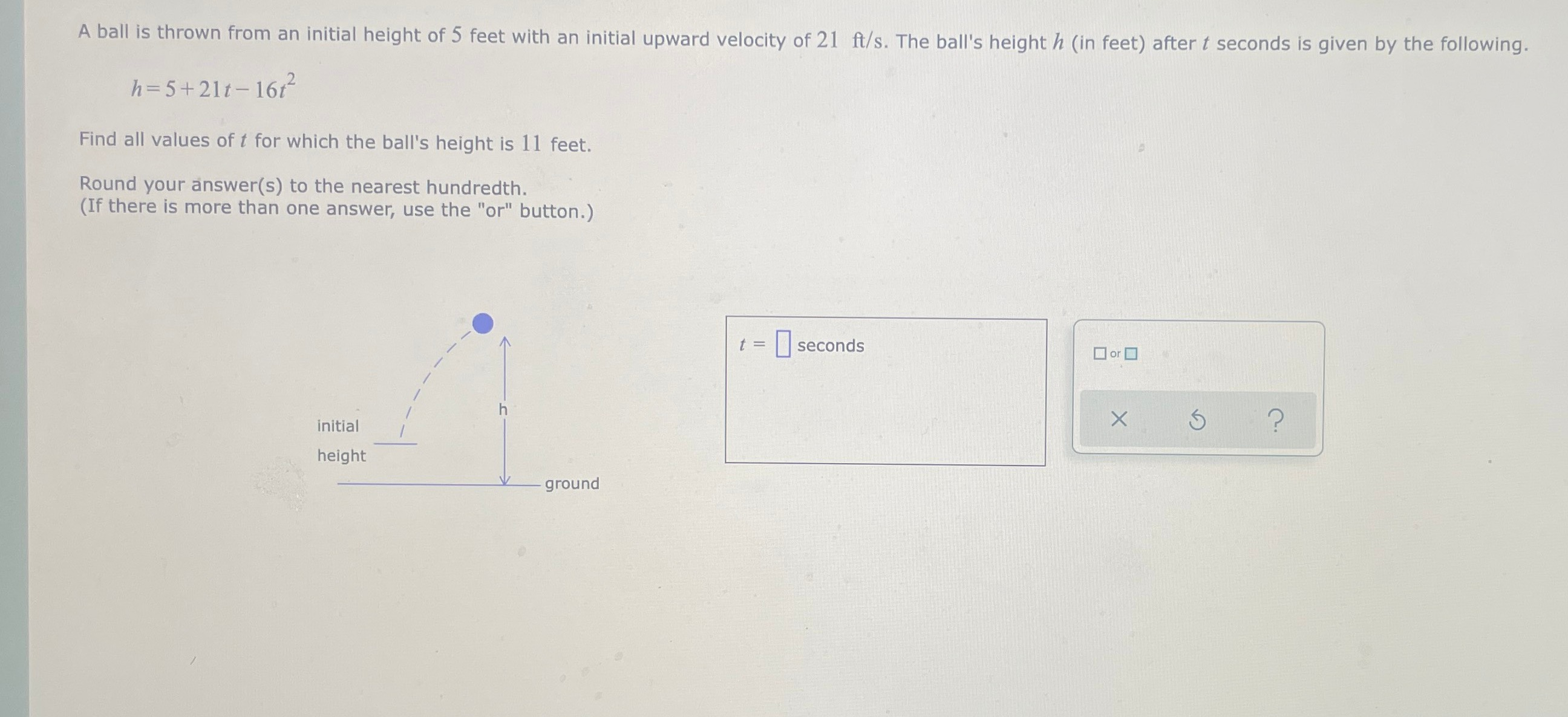### ¿Todavía tienes preguntas de matemáticas?

Pregunte a nuestros tutores expertos
Algebra
PreguntaA ball is thrown from an initial height of $$5$$ feet with an initial upward velocity of $$21 ft / s$$ . The ball's height $$h$$ (in feet) after $$t$$ seconds is given by the following.

How to solve

$$h = 5 + 21 t - 16 t ^ { 2 }$$

$$t = \square$$ seconds